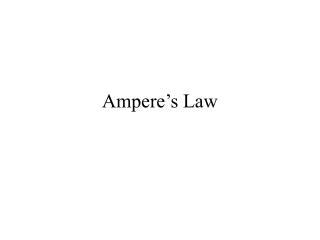DownloadDownload PresentationAmpere’s Law

Ampere’s Law

Télécharger la présentationAmpere’s Law

- - - - - - - - - - - - - - - - - - - - - - - - - - - E N D - - - - - - - - - - - - - - - - - - - - - - - - - - -
Presentation Transcript

1. Ampere’s Law

2. Outline • Introduce Ampere’s Law as an analogy to Gauss’ Law. • Define Ampere’s Law. • Show how to use Ampere’s Law for cases with symmetry.

3. Student Objectives • Recognise Ampere’s Law to be analogous to Gauss’ Law. • Recognise similar concepts: (1) draw an imaginary shape enclosing the current carrying conductor, (2) current enclosed.

4. Ampere’s Law • Gauss’ law allowed us to find the net electric field due to any charge distribution (with little effort) by applying symmetry. • Similarly the net magnetic field can be found with little effort if there is symmetry using Ampere’s law.

5. Ampere’s Law • Ampere’s law, • Where the integral is a line integral. • B.ds is integrated around a closed loop called an Amperian loop. • The current ienc is net current enclosed by the loop.

6. Ampere’s Law • ie, • ie ienc

7. Ampere’s Law • The figure shows the cross section of 3 arbitrary long straight wires with current as shown.

8. Ampere’s Law • Two of the currents are enclosed by an Amperian loop.

9. Direction of integration Ampere’s Law • An arbitrary direction for the integration is chosen.

10. Direction of integration Ampere’s Law • The loop is broken into elements of length ds (choose in the direction of the integration). • Direction of B doesn’t need to be known before the integration!

11. Direction of integration Ampere’s Law • B can be in an arbitrary direction at some angle to ds as shown (from the right hand grip rule we know B must in the plane of page). • We choose B to be in the direction as ds.

12. Ampere’s Law • The right hand screw (grip) rule is used to assign a direction to the enclosed currents. • A current passing through the loop in the same direction as the thumb are positive ( in the opposite direction -ve).

13. Direction of integration Ampere’s Law • Consider the integral,

14. Direction of integration Ampere’s Law • Applying the screw rule,

15. Ampere’s Law • Example. Find the magnetic field outside a long straight wire with current. I r

16. Amperian Loop Wire surface Ampere’s Law • We draw an Amperian loop and the direction of integration. Direction of Integration

17. Ampere’s Law • Recall, • Therefore, • The equation derived earlier.

18. Ampere’s Law • The positive sign for the current collaborates that the direction of B was correct.

19. Amperian Loop Wire surface Ampere’s Law • Example. Magnetic Field inside a Long Straight wire with current.

20. Ampere’s Law • Ampere’s Law,

21. Ampere’s Law • Ampere’s Law, • The charge enclosed is proportional to the area encircled by the loop,

22. Ampere’s Law • The current enclosed is positive from the right hand rule.